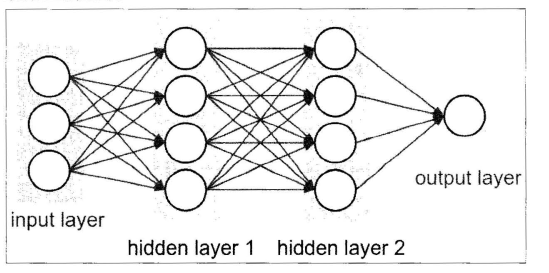## 【深度学习实战】从零开始深度学习（二）：多层全连接神经网络与MNIST手写数字分类

55
0
2021年1月10日 09时56分

1. 廖星宇《深度学习入门之PyTorch》
2. PyTorch官方文档
3. 其他参考资料在文中以超链接的方式给出

### 目录

• 0. 写在前面
• 1. PyTorch基础
• 1.1 张量（Tensor）
• 1.2 变量（Variable）
• 1.3 数据集（Dataset）
• 1.4 模组（nn.Module）
• 1.5 优化（torch.optim）
• 1.6 模型保存与加载
• 2. 案例实践：多层全连接神经网络实现 MNIST 手写数字分类
• 2.1 定义简单三层全连接神经网络
• 2.2 改进网络——增加激活函数
• 2.3 再改进一下网络——添加批标准化
• 2.4 训练网络
• 2.5 三个神经网络模型的比较

## 0. 写在前面

python （1）《笨方法学Python》（Learn Python the Hard Way）。这本书面向零基础的python学者，通过一系列简单的例子快速入门Python的基本操作。（2）廖雪峰的Python入门，这个系列教程可以更全面地学习Python，对于机器学习方向的同学来说掌握前几张的Python基础即可。（3）Edx: Introduction to Computer Science and Programming Using Python，这是MIT的公开课，以Python作为入门语言，简洁、全面地讲述了计算机科学的内容，适合更进一步的学习。

## 1. PyTorch基础

### 1.1 张量（Tensor）

a = torch.Tensor(([2,3], [4,8], [7,9]))
print('a is {}'.format(a))
print('a size is {}'.format(a.size()))
'''
out:
a is tensor([[2., 3.],
[4., 8.],
[7., 9.]])
a size is torch.Size([3, 2])
'''


a[0,1] = 100
print('a is changed to {}'.format(a))
'''
out:
a is changed to tensor([[  2., 100.],
[  4.,   8.],
[  7.,   9.]])
'''


numpy_a = a.numpy()
print('conver to numpy is \n {}'.format(numpy_a))
'''
out:
conver to numpy is
[[  2. 100.]
[  4.   8.]
[  7.   9.]]
'''

import numpy as np
b = np.array([[2,3], [4,5]])
torch_b = torch.from_numpy(b)
print('from numpy to torch.Tensor is {}'.format(torch_b))
'''
out:
from numpy to torch.Tensor is tensor([[2, 3],
[4, 5]], dtype=torch.int32)
'''


torch.Tensor 默认的是 torch.FloatTensor 数据类型，也可以定义我们想要的数据类型：

a = torch.LongTensor(([2,3], [4,8], [7,9]))
print('a is {}'.format(a))
'''
out:
a is tensor([[2, 3],
[4, 8],
[7, 9]])
'''


a = torch.zeros((3,2))
print('a is {}'.format(a))
'''
out:
a is tensor([[0., 0.],
[0., 0.],
[0., 0.]])
'''
a = torch.randn((3,2))
print('a is {}'.format(a))
'''
out:
a is tensor([[ 0.9284,  0.4900],
[ 0.3578, -1.0652],
[ 0.5255, -1.2100]])
'''


### 1.2 变量（Variable）

X = Variable(torch.Tensor ( [1) ) , requìres_grad=True)


1.3 数据集（Dataset）

• 继承和重写torch.utils.data.Dataset，例如：

import torch.utils.data.dataset as dataset
import pandas as pd

class myDataset(dataset):
def __init__(self, csv_file, txt_file, root_dir, other_file):
with open(txt_file, 'r') as f:
self.txt_data = data_list
self.root_dir = root_dir

def __len__(self):
return len(self.csv_data)

def __getitem__(self, idx):
data = (self.csv_data(idx), self.txt_data[idx])
return data


### 1.4 模组（nn.Module）

nn.Module是利用PyTorch建立神经网络的核心工具之一，神经网络中的层、损失函数都在这个包里面。所有模型的构建都是从nn.Module这个类继承来的。

### 1.5 优化（torch.optim）

• 一阶优化算法
最常用的一阶优化算法就是梯度下降。
• 二阶优化算法
二阶优化算法使用的是二阶导数，但是计算成本太高。torch.optim包提供了各种优化算法的实现，如随机梯度下降，以及添加动量的随机棉度下降，自适应学习率等。例如：

optimizer = torch.optim.SGD(model.parameters() , lr=0.01 , momentum=0.9 )
# 将模型的参数作为需要更新的参数传入优化器，设定学习率是 0.01 ，动量是 0.9 的随机梯度下降
optimizer.zeros() # 在优化之前需要先将梯度归零
loss.backward() # 反向传播，自动求导得到每个参数的梯度
optimizer.step() # 以通过梯度做一步参数更新

### 1.6 模型保存与加载

PyTorch里面提供了两种模型的保存方式，对应的也有两种模型的加载方式。

# 保存
torch.save(model, './model/pth')
# 加载


# 保存
torch.save(model.state_dict(), './model_state.pth')
# 加载


## 2. 案例实践：多层全连接神经网络实现 MNIST 手写数字分类

### 2.1 定义简单三层全连接神经网络

import torch.nn as nn

class simpleNet(nn.Module):
def __init__(self, in_dim, n_hidden_1, n_hidden_2, out_dim):
super(simpleNet, self).__init__()
self.layer1 = nn.Linear(in_dim, n_hidden_1)
self.layer2 = nn.Linear(n_hidden_1, n_hidden_2)
self.layer3 = nn.Linear(n_hidden_2, out_dim)

def forward(self, x):
x = self.layer1(x)
x = self.layer2(x)
x = self.layer3(x)
return x### 2.2 改进网络——增加激活函数

class Activation_Net(nn.Module):
def __init__(self, in_dim, n_hidden_1, n_hidden_2, out_dim):
super(Activation_Net, self).__init__()
self.layer1 = nn.Sequential(nn.Linear(in_dim, n_hidden_1),
nn.ReLU(True))
self.layer2 = nn.Sequential(nn.Linear(n_hidden_1, n_hidden_2),
nn.ReLU(True))
self.layer3 = nn.Sequential(nn.Linear(n_hidden_2, out_dim))

def forward(self, x):
x = self.layer1(x)
x = self.layer2(x)
x = self.layer3(x)
return x


### 2.3 再改进一下网络——添加批标准化

class Batch_Net(nn.Module):
def __init__(self, in_dim, n_hidden_1, n_hidden_2, out_dim):
super(Batch_Net, self).__init__()
self.layer1 = nn.Sequential(nn.Linear(in_dim, n_hidden_1),
nn.BatchNorm1d(n_hidden_1),
nn.ReLU(True))
self.layer2 = nn.Sequential(nn.Linear(n_hidden_1, n_hidden_2),
nn.BatchNorm1d(n_hidden_2),
nn.ReLU(True))
self.layer3 = nn.Sequential(nn.Linear(n_hidden_2, out_dim))

def forward(self, x):
x = self.layer1(x)
x = self.layer2(x)
x = self.layer3(x)
return x


### 2.4 训练网络

import torch
from torch import nn, optim
from torchvision import datasets, transforms

import net


# 设置超参数
batch_size = 64
learning_rate = 1e-2
num_epoches = 20


#数据预处理
data_tf = transforms.Compose([transforms.ToTensor(),
transforms.Normalize([0.5], [0.5])])


torchvision.transforms提供了很多图片的预处理方法。这里的transforms.ToTensor()将图片转换成PyTorch中处理的Tensor对象，在转换的过程中PyTorch自动将图片标准化了；transforms.Normalize()需要传入两个参数，第一个参数市均值，第二个参数是方差，做的处理就是减均值，再除以方差。transforms.Compose()将各种预处理操作组合在一起。

#下载训练集-MNIST手写数字训练集
test_dataset = datasets.MNIST(root="./data", train=False, transform=data_tf)


model = net.simpleNet(28*28, 300, 100, 10)
if torch.cuda.is_available():
model = model.cuda()
criterion = nn.CrossEntropyLoss()
optimizer = optim.SGD(model.parameters(), lr = learning_rate)


# 训练模型
def train_model(model, criterion, optimizer, num_epoches):
for epoch in range(num_epoches):
print('epoch {}/{}'.format(epoch, num_epoches-1))
print('-'*10)
## training------------------
#        model.train()
train_loss = 0.0
train_acc = 0.0
# 获取数据输入和标签，封装成变量
img, label = data # 获得图片和标签
img = img.view(img.size(0), -1) #将图片进行img的转换
if torch.cuda.is_available():
img = Variable(img).cuda()
label = Variable(label).cuda()
else:
img, label = Variable(img), Variable(label)
# 梯度参数清零
# 前向
out = model(img) # 等价于 out = model.forward(img)
loss = criterion(out, label)
_, preds = torch.max(out.data, 1)
# 反向传播
loss.backward()
optimizer.step()
# 统计
train_loss += loss.item()
train_correct = torch.sum(preds == label.data)
train_acc += train_correct

## evaluation-------------
model.eval()
eval_loss = 0.0
eval_acc = 0.0
img, label = data
img = img.view(img.size(0), -1)
if torch.cuda.is_available():
img = Variable(img).cuda()
label = Variable(label).cuda()
else:
img = Variable(img, volatile = True)
label = Variable(label, volatile = True)
out = model(img)
loss = criterion(out, label)
eval_loss += loss.item()
_, preds = torch.max(out.data, 1)
num_correct = torch.sum(preds == label.data)
eval_acc += num_correct


import torch

a = torch.Tensor(2,3)
print(a)
# tensor([[0.0000, 0.0000, 0.0000],
#        [0.0000, 0.0000, 0.0000]])

print(a.view(1,-1))
# tensor([[0.0000, 0.0000, 0.0000, 0.0000, 0.0000, 0.0000]])


train_model(model, criterion, optimizer, num_epoches)


### 2.5 三个神经网络模型的比较